LTE Quick Reference

PSS (Primary Synchronization Channel)

PSS is a specific physical layer signal that is used for radio frame synchronization. It has characterstics as listed below.

• Mapped to 72 active sub carriers(6 resource blocks), centered around the DC subcarrier in slot 0 (Subframe 0) and slot 10 (Subframe 5) in FDD.
• Mapped to 72 active sub carriers(6 resource blocks), centered around the DC subcarrier in slot 2 (Subframe 2) and slot 12 (Subframe 6) in TDD.
• Made up of 62 Zadoff Chu Sequence Values
• Used for Downlink Frame Synchronization
• One of the critical factors determining Physical Cell ID

May Not be a big issues for most of the case since it would be working fine for most of the device that you have for test. Otherwise it would have not been given to you for test.

However, If you are a developer working at early stage of LTE chipset, this would be one of the first signal you have to implement.

Algorithm for PSS Generation

The exact PSS symbol calculation is done by the following formula as described in 36.211 - 6.11.1. For the specific example of generated PSS, refer to Matlab : Toolbox : LTE : PSS page or PSS with default Matlab function.Generating PSS with default Matlab function

Followings are the Matlab code for generating PSS and its result for each NID value.

clear all;

u_shift = [25 29 34];

NID = 0;

d_u = [];

for n = 0:61

u = u_shift(NID+1);

if n <= 30

d = exp(-j*pi*u*n*(n+1)/63);

else

d = exp(-j*pi*u*(n+1)*(n+2)/63);

end;

d_u = [d_u d];

end;

subplot(1,3,1);

plot(real(d_u(1:31)),imag(d_u(1:31)),'ko','MarkerFaceColor',[0 0 0]);

axis([-1.5 1.5 -1.5 1.5]);

title('n=0..30');

subplot(1,3,2);

plot(real(d_u(32:62)),imag(d_u(32:62)),'bo','MarkerFaceColor',[0 0 1]);

axis([-1.5 1.5 -1.5 1.5]);

title('n=31..61');

subplot(1,3,3);

plot(real(d_u(1:62)),imag(d_u(1:62)),'ro','MarkerFaceColor',[1 0 0]);

axis([-1.5 1.5 -1.5 1.5]);

title('n=0..61');

 NID Result 0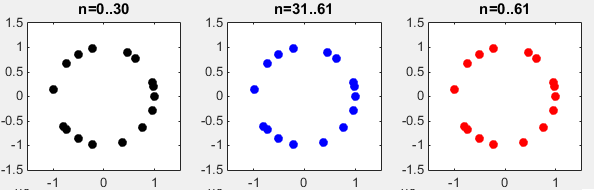12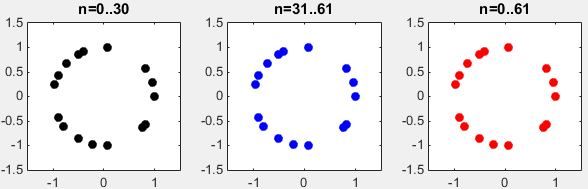Followings are the numberical result (print out of d_u[ ] array) for each NID;

 NID = 0 NID = 1 NID = 2 1.0000 + 0.0000i   -0.7971 - 0.6038i    0.3653 - 0.9309i   -0.7331 - 0.6802i    0.9802 + 0.1981i    0.9556 + 0.2948i   -0.5000 - 0.8660i    0.7660 - 0.6428i   -0.2225 - 0.9749i    0.6235 + 0.7818i    0.4562 + 0.8899i    0.3653 - 0.9309i    0.9556 + 0.2948i    0.7660 - 0.6428i   -0.5000 + 0.8660i   -0.7331 + 0.6802i    0.9802 + 0.1981i   -0.2225 + 0.9749i    0.6235 + 0.7818i   -0.7971 - 0.6038i   -0.5000 - 0.8660i   -0.5000 + 0.8660i   -0.7971 - 0.6038i   -0.9888 + 0.1490i    0.9556 - 0.2948i    0.9802 + 0.1981i   -0.2225 - 0.9749i    1.0000 - 0.0000i    0.7660 - 0.6428i   -0.7331 + 0.6802i   -0.9888 + 0.1490i   -0.9888 + 0.1490i   -0.7331 + 0.6802i    0.7660 - 0.6428i    1.0000 - 0.0000i   -0.2225 - 0.9749i    0.9802 + 0.1981i    0.9556 - 0.2948i   -0.9888 + 0.1490i   -0.7971 - 0.6038i   -0.5000 + 0.8660i   -0.5000 - 0.8660i   -0.7971 - 0.6038i    0.6235 + 0.7818i   -0.2225 + 0.9749i    0.9802 + 0.1981i   -0.7331 + 0.6802i   -0.5000 + 0.8660i    0.7660 - 0.6428i    0.9556 + 0.2948i    0.3653 - 0.9309i    0.4562 + 0.8899i    0.6235 + 0.7818i   -0.2225 - 0.9749i    0.7660 - 0.6428i   -0.5000 - 0.8660i    0.9556 + 0.2948i    0.9802 + 0.1981i   -0.7331 - 0.6802i    0.3653 - 0.9309i   -0.7971 - 0.6038i    1.0000 + 0.0000i 1.0000 + 0.0000i   -0.9691 - 0.2468i   -0.7331 - 0.6802i    0.0747 + 0.9972i   -0.7971 + 0.6038i    0.8262 + 0.5633i   -0.5000 + 0.8660i    0.7660 + 0.6428i   -0.9010 + 0.4339i   -0.2225 + 0.9749i   -0.4113 - 0.9115i   -0.7331 - 0.6802i    0.8262 + 0.5633i    0.7660 + 0.6428i   -0.5000 - 0.8660i    0.0747 - 0.9972i   -0.7971 + 0.6038i   -0.9010 - 0.4339i   -0.2225 + 0.9749i   -0.9691 - 0.2468i   -0.5000 + 0.8660i   -0.5000 - 0.8660i   -0.9691 - 0.2468i    0.9556 - 0.2948i    0.8262 - 0.5633i   -0.7971 + 0.6038i   -0.9010 + 0.4339i    1.0000 - 0.0000i    0.7660 + 0.6428i    0.0747 - 0.9972i    0.9556 - 0.2948i    0.9556 - 0.2948i    0.0747 - 0.9972i    0.7660 + 0.6428i    1.0000 + 0.0000i   -0.9010 + 0.4339i   -0.7971 + 0.6038i    0.8262 - 0.5633i    0.9556 - 0.2948i   -0.9691 - 0.2468i   -0.5000 - 0.8660i   -0.5000 + 0.8660i   -0.9691 - 0.2468i   -0.2225 + 0.9749i   -0.9010 - 0.4339i   -0.7971 + 0.6038i    0.0747 - 0.9972i   -0.5000 - 0.8660i    0.7660 + 0.6428i    0.8262 + 0.5633i   -0.7331 - 0.6802i   -0.4113 - 0.9115i   -0.2225 + 0.9749i   -0.9010 + 0.4339i    0.7660 + 0.6428i   -0.5000 + 0.8660i    0.8262 + 0.5633i   -0.7971 + 0.6038i    0.0747 + 0.9972i   -0.7331 - 0.6802i   -0.9691 - 0.2468i    1.0000 + 0.0000i 1.0000 + 0.0000i   -0.9691 + 0.2468i   -0.7331 + 0.6802i    0.0747 - 0.9972i   -0.7971 - 0.6038i    0.8262 - 0.5633i   -0.5000 - 0.8660i    0.7660 - 0.6428i   -0.9010 - 0.4339i   -0.2225 - 0.9749i   -0.4113 + 0.9115i   -0.7331 + 0.6802i    0.8262 - 0.5633i    0.7660 - 0.6428i   -0.5000 + 0.8660i    0.0747 + 0.9972i   -0.7971 - 0.6038i   -0.9010 + 0.4339i   -0.2225 - 0.9749i   -0.9691 + 0.2468i   -0.5000 - 0.8660i   -0.5000 + 0.8660i   -0.9691 + 0.2468i    0.9556 + 0.2948i    0.8262 + 0.5633i   -0.7971 - 0.6038i   -0.9010 - 0.4339i    1.0000 + 0.0000i    0.7660 - 0.6428i    0.0747 + 0.9972i    0.9556 + 0.2948i    0.9556 + 0.2948i    0.0747 + 0.9972i    0.7660 - 0.6428i    1.0000 + 0.0000i   -0.9010 - 0.4339i   -0.7971 - 0.6038i    0.8262 + 0.5633i    0.9556 + 0.2948i   -0.9691 + 0.2468i   -0.5000 + 0.8660i   -0.5000 - 0.8660i   -0.9691 + 0.2468i   -0.2225 - 0.9749i   -0.9010 + 0.4339i   -0.7971 - 0.6038i    0.0747 + 0.9972i   -0.5000 + 0.8660i    0.7660 - 0.6428i    0.8262 - 0.5633i   -0.7331 + 0.6802i   -0.4113 + 0.9115i   -0.2225 - 0.9749i   -0.9010 - 0.4339i    0.7660 - 0.6428i   -0.5000 - 0.8660i    0.8262 - 0.5633i   -0.7971 - 0.6038i    0.0747 - 0.9972i   -0.7331 + 0.6802i   -0.9691 + 0.2468i    1.0000 - 0.0000i

Threre are three types of PSSs in LTE. To make all these three type unique (distinctive to each other), it is designed that the cross correlation between each PSS be very low. Following example shows the cross correlation between each PSS.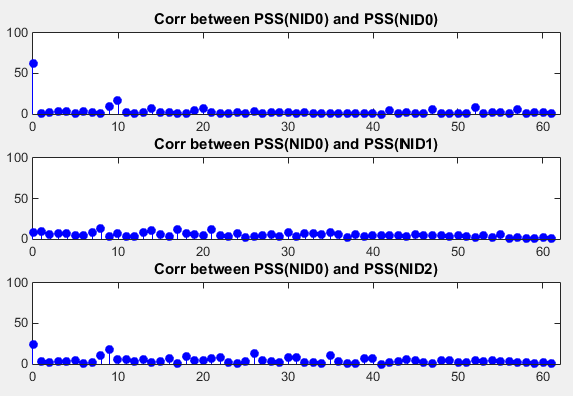Following is the Matlab source code that produce the result as shown above. I used Matlab dsp package to calculate the cross correlation. If you don't have Matlab dsp package, try to write your own script for calculating cross correlation

clear all;

u_shift = [25 29 34];

% Generate PSS for NID = 0

NID = 0;

d_u = [];

for n = 0:61

u = u_shift(NID+1);

if n <= 30

d = exp(-j*pi*u*n*(n+1)/63);

else

d = exp(-j*pi*u*(n+1)*(n+2)/63);

end;

d_u = [d_u d];

end;

d_u_NID0 = d_u';

% Generate PSS for NID = 1

NID = 1;

d_u = [];

for n = 0:61

u = u_shift(NID+1);

if n <= 30

d = exp(-j*pi*u*n*(n+1)/63);

else

d = exp(-j*pi*u*(n+1)*(n+2)/63);

end;

d_u = [d_u d];

end;

d_u_NID1 = d_u';

% Generate PSS for NID = 2

NID = 2;

d_u = [];

for n = 0:61

u = u_shift(NID+1);

if n <= 30

d = exp(-j*pi*u*n*(n+1)/63);

else

d = exp(-j*pi*u*(n+1)*(n+2)/63);

end;

d_u = [d_u d];

end;

d_u_NID2 = d_u';

% Cross Correlation between PSS

Hxcorr = dsp.Crosscorrelator;

taps = 0:61;

taps = taps';

XCorr_0_0 = step(Hxcorr,d_u_NID0,d_u_NID0);

XCorr_0_1 = step(Hxcorr,d_u_NID0,d_u_NID1);

XCorr_0_2 = step(Hxcorr,d_u_NID0,d_u_NID2);

subplot(3,1,1);

stem(taps,abs(XCorr_0_0(62:end)),'bo','MarkerFaceColor',[0 0 1]);

xlim([0 length(taps)]); ylim([0 100]);

title('Corr between PSS(NID0) and PSS(NID1)');

subplot(3,1,2);

stem(taps,abs(XCorr_0_1(62:end)),'bo','MarkerFaceColor',[0 0 1]);

xlim([0 length(taps)]); ylim([0 100]);

title('Corr between PSS(NID0) and PSS(NID2)');

subplot(3,1,3);

stem(taps,abs(XCorr_0_2(62:end)),'bo','MarkerFaceColor',[0 0 1]);

xlim([0 length(taps)]); ylim([0 100]);

title('Corr between PSS(NID0) and PSS(NID3)');

Correlation between a PSS and its PhaseShifted Version

This example shows the correlation between a PSS and its phaseshifted copy. As you see here, the magnitue (absolute value) of correlation does not changes even if you do phase shift. However, you can figure out the degree of phase shift by taking the angle of correlation.

With this property, you can identify a PSS in a received signal without worrying about any possible phase shift that might have occurred by communication channel. In addition, you can figure out the amount of phase shift by taking the angle value of the correlation and use that value to compensate (undo) the phase shift.

 Correlation between two identical PSS Correlation between a PSS and pi/3 rad shifted version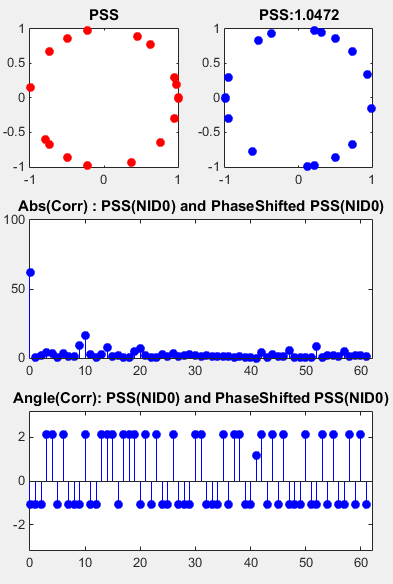Following is the matlab source code that produced the result shown above. I used Matlab dsp package to calculate the cross correlation. If you don't have Matlab dsp package, try to write your own script for calculating cross correlation

clear all;

u_shift = [25 29 34];

% Generate PSS for NID = 0

NID = 0;

d_u = [];

for n = 0:61

u = u_shift(NID+1);

if n <= 30

d = exp(-j*pi*u*n*(n+1)/63);

else

d = exp(-j*pi*u*(n+1)*(n+2)/63);

end;

d_u = [d_u d];

end;

phShift = pi/3;

d_u_NID0 = transpose(d_u); % Original PSS

d_u_NID0_PhaseShift = transpose(d_u .* exp(j*phShift)); % PhaseShifted PSS

% Cross Correlation between PSS

Hxcorr = dsp.Crosscorrelator;

taps = 0:61;

taps = taps';

XCorr_0_0_Shifted = step(Hxcorr,d_u_NID0,d_u_NID0_PhaseShift);

subplot(3,2,1);

plot(real(d_u_NID0),imag(d_u_NID0),'ro','MarkerFaceColor',[1 0 0]);

title('PSS');

subplot(3,2,2);

plot(real(d_u_NID0_PhaseShift),imag(d_u_NID0_PhaseShift),'bo','MarkerFaceColor',[0 0 1]);

title(strcat('PSS:',num2str(phShift)));

subplot(3,2,[3 4]);

stem(taps,abs(XCorr_0_0_Shifted(62:end)),'bo','MarkerFaceColor',[0 0 1]);

xlim([0 length(taps)]); ylim([0 100]);

title('Abs(Corr) : PSS(NID0) and PhaseShifted PSS(NID0)');

subplot(3,2,[5 6]);

stem(taps,angle(XCorr_0_0_Shifted(62:end)),'bo','MarkerFaceColor',[0 0 1]);

xlim([0 length(taps)]); ylim([-pi pi]);

title('Angle(Corr): PSS(NID0) and PhaseShifted PSS(NID0)');

Correlation between a PSS and its Noised Version

This example shows the noise tolerance of PSS. The example on the left shows the correlation between a PSS and the one with 50 dB SNR (practically no Noise condition) and the example on the right shows the correlation between a PSS and the one with 10 dB SNR.

 Correlation between two identical PSS Correlation between a PSS and noised version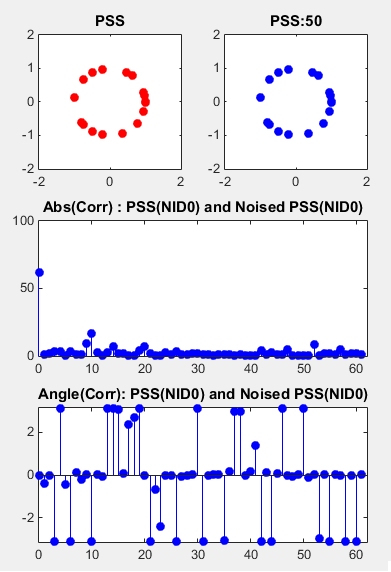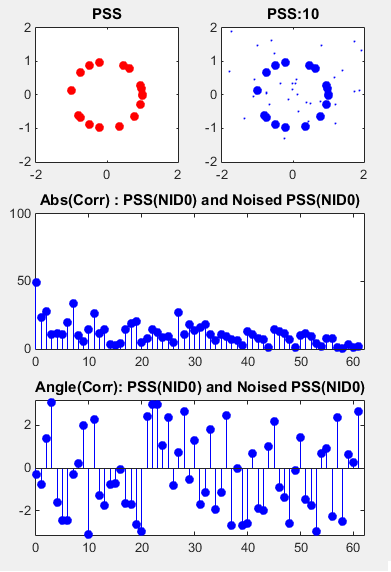Following is the matlab source code that produced the result shown above. I used Matlab dsp package to calculate the cross correlation. If you don't have Matlab dsp package, try to write your own script for calculating cross correlation.

clear all;

u_shift = [25 29 34];

% Generate PSS for NID = 0

NID = 0;

d_u = [];

for n = 0:61

u = u_shift(NID+1);

if n <= 30

d = exp(-j*pi*u*n*(n+1)/63);

else

d = exp(-j*pi*u*(n+1)*(n+2)/63);

end;

d_u = [d_u d];

end;

d_u_NID0 = transpose(d_u);

% Specify SNR in dB. Try setting various different value here and see how the result changes

SNR_dB = 10;

% Get the number of symbols

N = length(d_u_NID0);

% Calculate Symbol Energy

Eavg = sum(abs(d_u_NID0) .^ 2)/length(N);

% Convert SNR (in dB) to SNR (in Linear)

SNR_lin = 10 .^ (SNR_dB/10);

% Calculate the Sigma (Standard Deviation) of AWGN

awgnSigma = sqrt(Eavg/(2*SNR_lin));

% Generate a sequence of noise with Normal Distribution and rescale it with the sigma

awgn = awgnSigma*(randn(1,N)+j*randn(1,N));

awgn = awgn';

% Add AWGN to PSS

d_u_NID0_Awgn = d_u_NID0 + awgn;

% Cross Correlation between PSS

Hxcorr = dsp.Crosscorrelator;

taps = 0:61;

taps = taps';

XCorr_0_0_AWGN = step(Hxcorr,d_u_NID0,d_u_NID0_Awgn);

subplot(3,2,1);

plot(real(d_u_NID0),imag(d_u_NID0),'ro','MarkerFaceColor',[1 0 0]);

axis([-2 2 -2 2]);

title('PSS');

subplot(3,2,2);

plot(real(d_u_NID0),imag(d_u_NID0),'bo', ...

'MarkerFaceColor',[0 0 1]);

hold on;

plot(real(d_u_NID0_Awgn),imag(d_u_NID0_Awgn),'bo', ...

'MarkerFaceColor',[0 0 0],'MarkerSize',1);

axis([-2 2 -2 2]);

title(strcat('PSS:',num2str(SNR_dB)));

hold off;

subplot(3,2,[3 4]);

stem(taps,abs(XCorr_0_0_AWGN(62:end)),'bo','MarkerFaceColor',[0 0 1]);

xlim([0 length(taps)]); ylim([0 100]);

title('Abs(Corr) : PSS(NID0) and Noised PSS(NID0)');

subplot(3,2,[5 6]);

stem(taps,angle(XCorr_0_0_AWGN(62:end)),'bo','MarkerFaceColor',[0 0 1]);

xlim([0 length(taps)]); ylim([-pi pi]);

title('Angle(Corr): PSS(NID0) and Noised PSS(NID0)');

Reference :